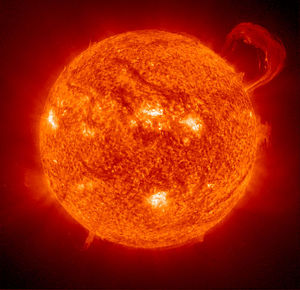# Mass-energy equivalenceFigure 1. The sun produces its energy by the conversion of mass into energy through the process of nuclear fusion.

Mass-energy equivalence is the famous concept in physics represented mathematically by $E=mc^{2}$, which states that mass and energy are one and the same. This idea was not actually put forth by Einstein, but he was the first to describe an accurate relationship for it in his theory of special relativity, where he first wrote down this famous equation. The $c^{2}$term is a tremendously large quantity, so this means that a small amount of mass corresponds to a large amount of energy. This equation is only representative of an object at rest, so this energy is called the "rest energy" of an object. The full equation Einstein wrote down includes the energy of a moving object, but the simplified version is still profound.

The implications of such an idea are overwhelming. Mass can be created out of energy, it just takes a lot of energy to do this. In fact, the entire universe was born in the Big Bang when a whole lot of energy was turned into mass.

For example, burning a gallon of gasoline (3.78 liters) releases about 132 million joules of energy, which is enough energy to make 14 ng of mass. This is roughly the mass of a single particle of very finely ground flour. No scale in the world can detect a difference of 14 ng out of the 3 kg of mass of the gasoline.

This naturally leads to the conclusion that 'if the technology existed to just turn that gasoline into pure energy', the world's energy problems would go away. Unfortunately, this is forbidden by a deep physical law that says the total number of protons and neutrons must remain the same. Protons can become neutrons, and neutrons can become protons (and both happen with beta decay). This law is known as baryon conservation and is discussed at hyperphysics (both protons and neutrons are baryons).

In the gasoline case, a gallon of gasoline weighs about 3 kilograms (~6 pounds). The loss of a nanogram is impossible to detect with any scale, so that's all theoretical.

The strong force, weak force and electromagnetic force work together inside of a nucleus to create stable configurations of protons and neutrons. These nuclear processes make for much stronger forces than the electron recombination in the combustion of fossil fuels does. This means that the release of energy from a nuclear reaction creates enough of a mass difference to be measured. Specifically, small amounts of mass are turned into energy from the breaking up (fission) or combination (fusion) of the nuclei of atoms. Even spontaneous radioactive decay converts a bit of mass into incredible amounts of energy.

By doing so, the energy from these processes can be used to generate electricity in nuclear power plants, or as nuclear weapons, which were first deployed in World War II and have only been tested since then (nuclear weapons have since not been used as a direct attack, just very big threats). Although these reactions cannot convert the entire mass to energy, they still release tremendous amounts of energy. See the page on energy density for more information.

The sun uses fusion of hydrogen into helium to create sunlight at an astonishing rate. The sun gives off 3.86 x 1026 W of power. That means the sun is losing 4.2 million tonnes of mass every second due to nuclear fusion.